#2019 iT 邦幫忙鐵人賽DAY 6
0

## 【Day 6】 使用JavaScript 做字串處理

``````msg = "Hello," + ",ithome"
console.log(msg);
``````

-> Hello,ithome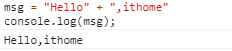``````var i = 10;
var j = 15;
var sum = i + j ;
console.log(sum);
``````

->25``````var s = "Hello, ithome"
s.length //s 字串長度
``````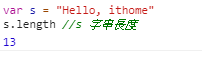``````s.charAt(0); //s 第一個字元
``````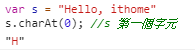``````s.charAt(s.length-1) //最後一個字元
``````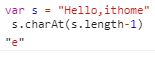``````s.substring(0,5) //從索引0，取5個字元  -> Hello
``````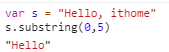``````s.slice(-6) //取倒數6個字元
``````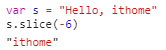``````s.indexOf("H") // 取H字母 在字符串中首次出現的位置
``````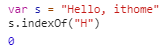``````s.replace("o","O") //用"O" 取代"o" ->這邊發現無法完全取代o， 因此要加上正規表達式，改成
``````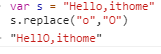``````s.replace(/o/g,"O")
``````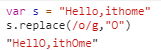``````s.toUpperCase() //全部字串轉大寫
``````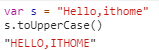``````s.toLowerCase() //全部字串轉小寫
``````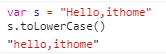JavaScript大全
https://www.books.com.tw/products/0010542183

https://coder.tw/?p=7258